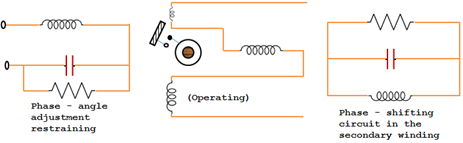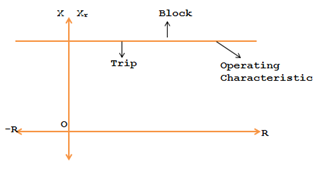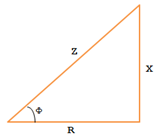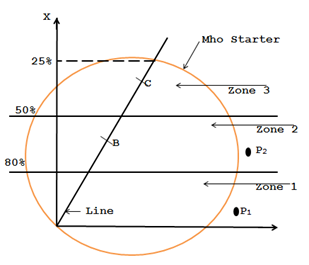# Induction Type Reactance Relay

A simplest form of electromagnetic induction type reactance relay (Induction Cup) is shown in the figure. The current is the operating quantity. It produces flux in the upper, lower and right hand side poles. The right hand side pole flux is out of phase with the flux in the lower and upper poles because of the secondary winding which is closed by a phase shifting circuit and is placed on the right hand side pole. The polarizing flux and the right hand side pole flux interacts to produce the operating torque K1I2. The interaction of left hand side pole flux and the polarizing flux produces the restraining torque. The phase angle adjustment circuit is connected in series with the voltage coil.A reactance relay measures the reactance of the line at the relay location. This induction type reactance relay performance is not affected by arc resistance during the occurrence of the fault. In case of a fault, the relay measures the reactance of the line up to the fault point from the relay location.

### R-X Diagram of Induction Type Reactance Relay

The relays operating characteristic on R-X diagram, is a straight line parallel to X axis as indicated below in figure.The operating torque is by current and the Restraining torque is by current–voltage directional element. The Reactance relay may also be called as over current relay with directional restraint. The directional element is arranged to develop maximum negative torque, when the current lags its voltage by 90o. If the spring control effect is K3, the torque T is given by:

T = K1l2 – K2VI sin Φ – K3        where sin Φ = Cos (90 – Φ)

As the value of K3 is very small, it can be neglected.
T = K1l2 – K2VI sin Φ.
VIsinΦ indicates reactive voltamperes.As the start of relay operation,
K1l2 = K2VIsinΦ
K1I = K2 V SinΦ
(V/I) = K1 / K2 sinΦ
Z sinΦ = K1/K2 = X = Constant

Operating torque will be more than the Restraining torque for relay operation. In other words the Restraining torque should be less than the operating torque.

i.e., K2 VIsinΦ < K1I2
K2VsinΦ < K1I
V/I sinΦ < K1/K2 < K, a constant
X < K, as  X = Z sinΦ and Z = V/I

The reactance relay will operate, when the measured value of the reactance is less than the predetermined or design value of K.

The directional unit used with the reactance relay is not the same as the one used with the impedance type relay because the reactance relay will trip under normal load conditions when the power factor of the load is unity or near zero. This is because; the restraining reactive volt-ampere at U.P.F or near U.P.F will be near zero. Therefore, we must have directional unit is called Mho unit or Mho relay, having a circular characteristic.

Reactance relay are used for protection of short lines having fault currents less than 20 KA. In such lines, the effect of fault resistance or arc resistance is predominant.

### Reactance Relay With MHO Starter

For first, second and third zone protection of the transmission line, reactance relays as employs for 1st and 2nd zones. The directional unit, a mho starter relay acts as 3rd zone protective relay.The zone I unit is a high speed unit to protect 80 % to 90% of the protected line. The second unit protects up to 50% of the adjacent line. The 3rd unit is a back-up unit, to protect the whole of the adjacent line.

The directional unit used with the reactance relay, viz. mho starter, has a circular characteristic, because of the reason explained below:

Under normal conditions, with a load of high power factor the reactance measured by the relay may be less than its setting. Such points P1 and P2 in the diagram lie in zone 1 and 2 respectively. To prevent false tripping under such conditions, the reactance relay should be supervised by a fault detecting unit (starting unit or starter), which limits its area on the R-X diagram. Hence its characteristic should be a circular one. The starting unit detects fault and also serves the function of 3rd zone unit.0 CommentsComments